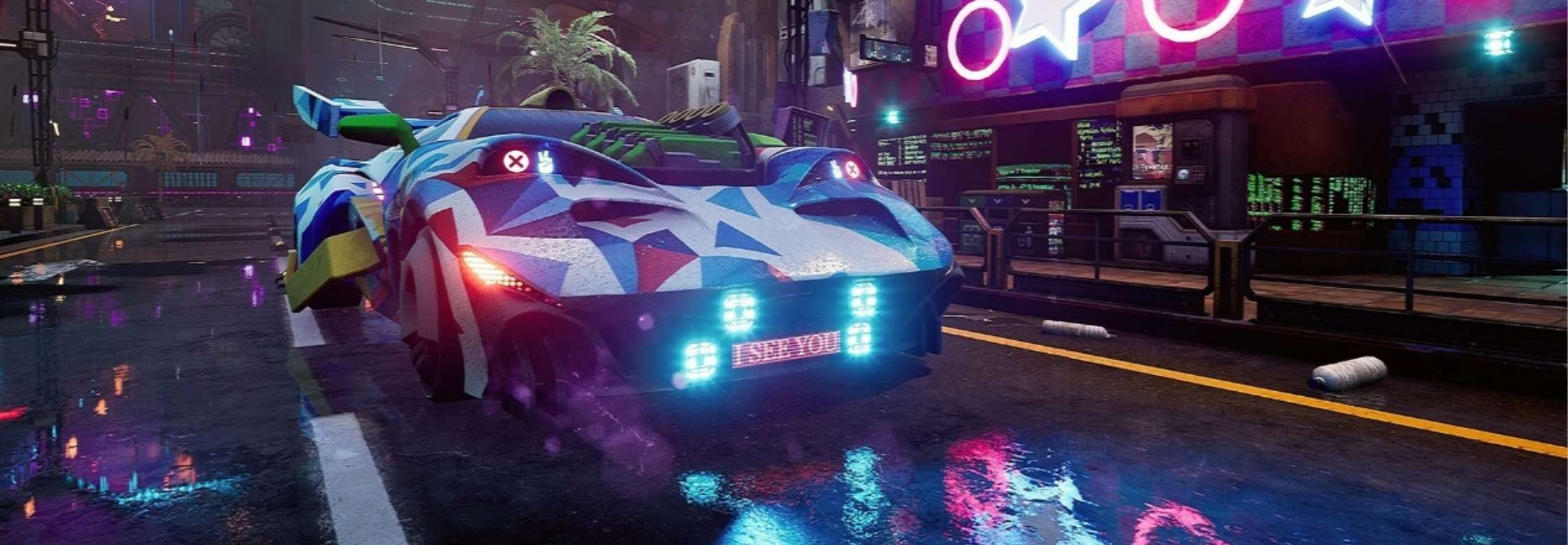# 高品质后处理：十种图像模糊算法的总结与实现

• 高斯模糊（Gaussian Blur）
• 方框模糊（Box Blur）
• Kawase模糊（Kawase Blur）
• 双重模糊（Dual Blur）
• 散景模糊（Bokeh Blur）
• 移轴模糊（Tilt Shift Blur）
• 光圈模糊（Iris Blur）
• 粒状模糊（Grainy Blur）
• 方向模糊（Directional Blur）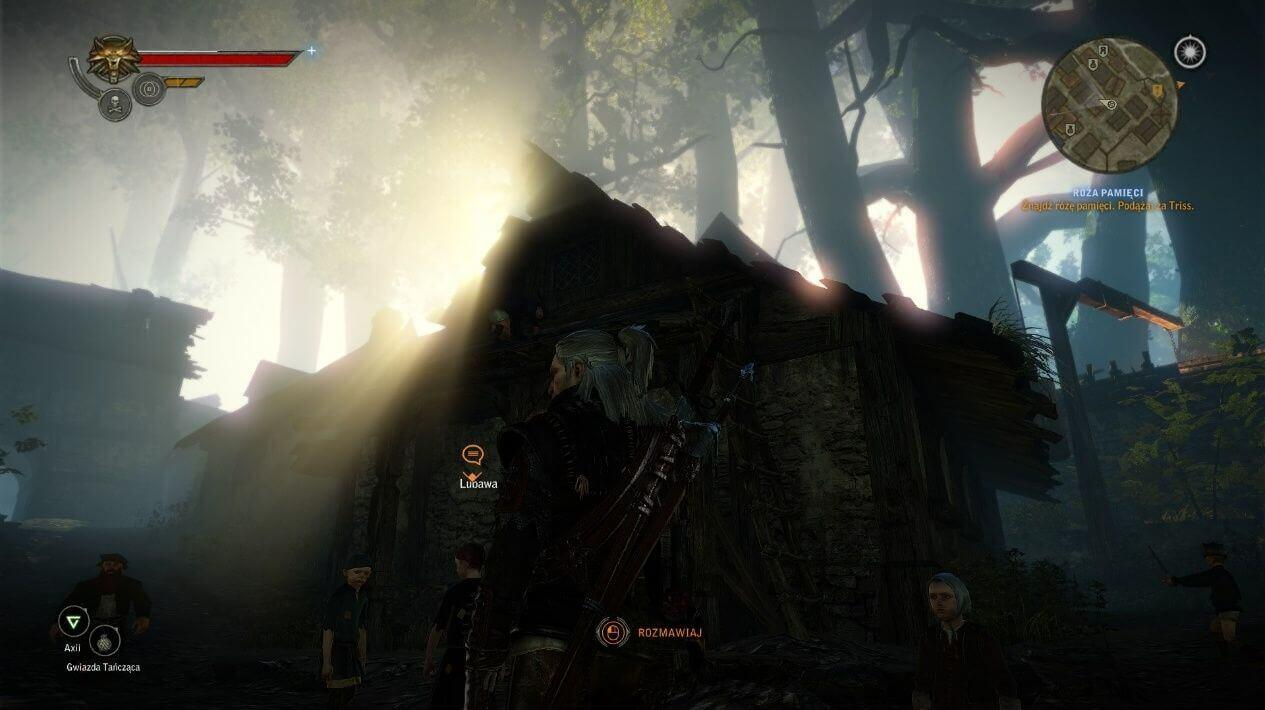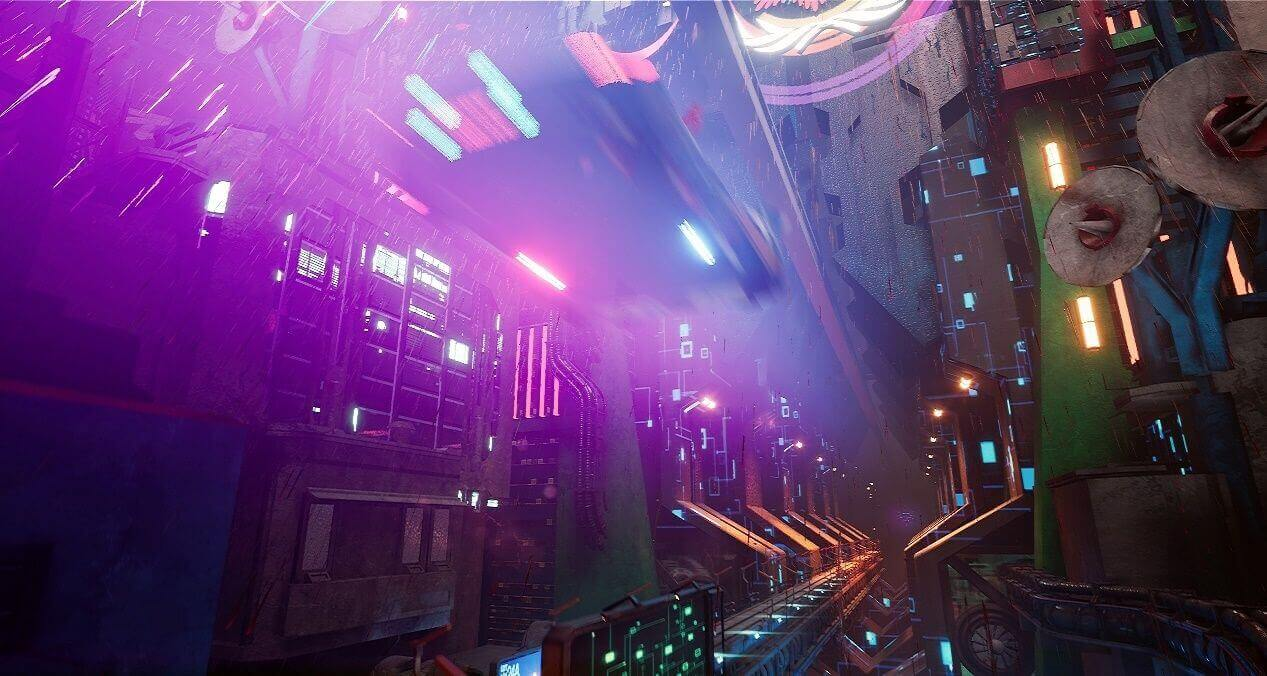UE4场景中，间接基于高斯模糊（Gaussian Blur）的Bloom特效给画面带了更好的光感 @ UE4 CyberNeon @Junliang Zhang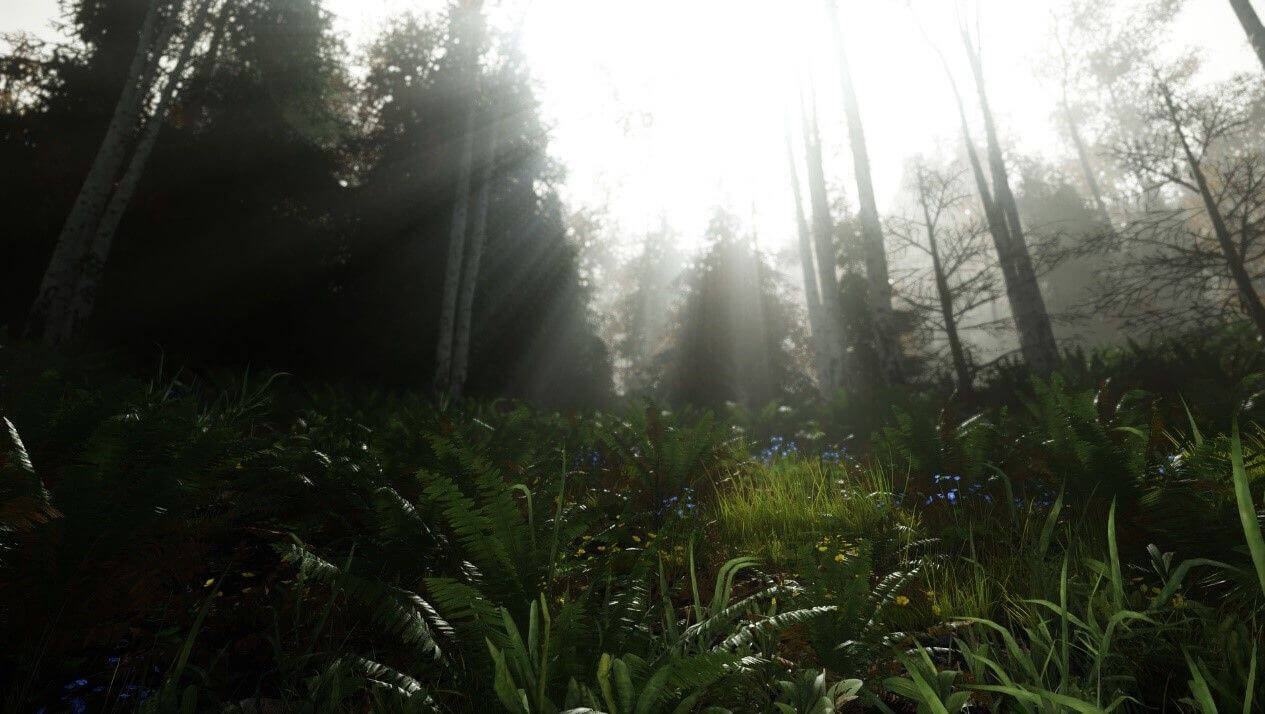Sun Shaft Forest @UE4《鬼泣5》中的镜头眩光光晕（Glare Lens Flare）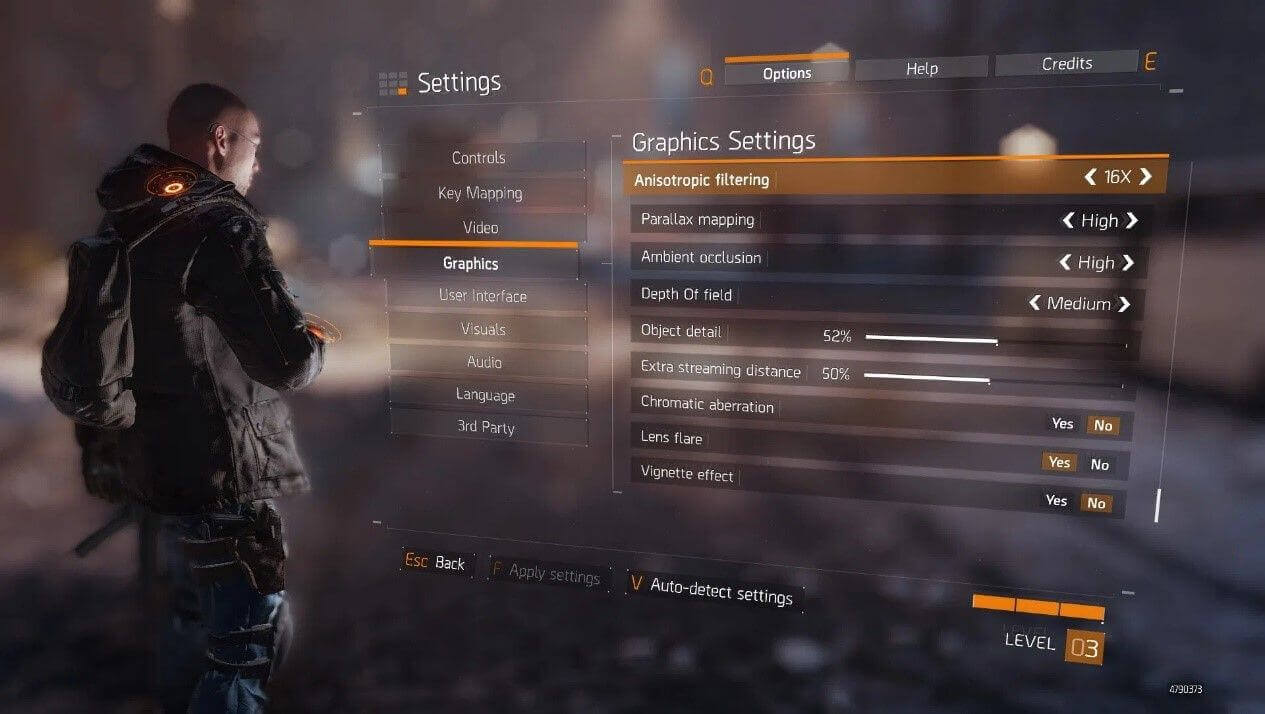Tom Clancys The Division中的基于散景模糊（Bokeh Blur）的景深

### 十种图像模糊算法横向对比

• 模糊品质（Quality）：模糊品质的好坏是模糊算法是否优秀的主要指标。
• 模糊稳定性（Stability）：模糊的稳定性决定了在画面变化过程中，模糊是否稳定，不会出现跳变或者闪烁。
• 性能（Performance）：性能的好坏是模糊算法是否能被广泛使用的关键所在。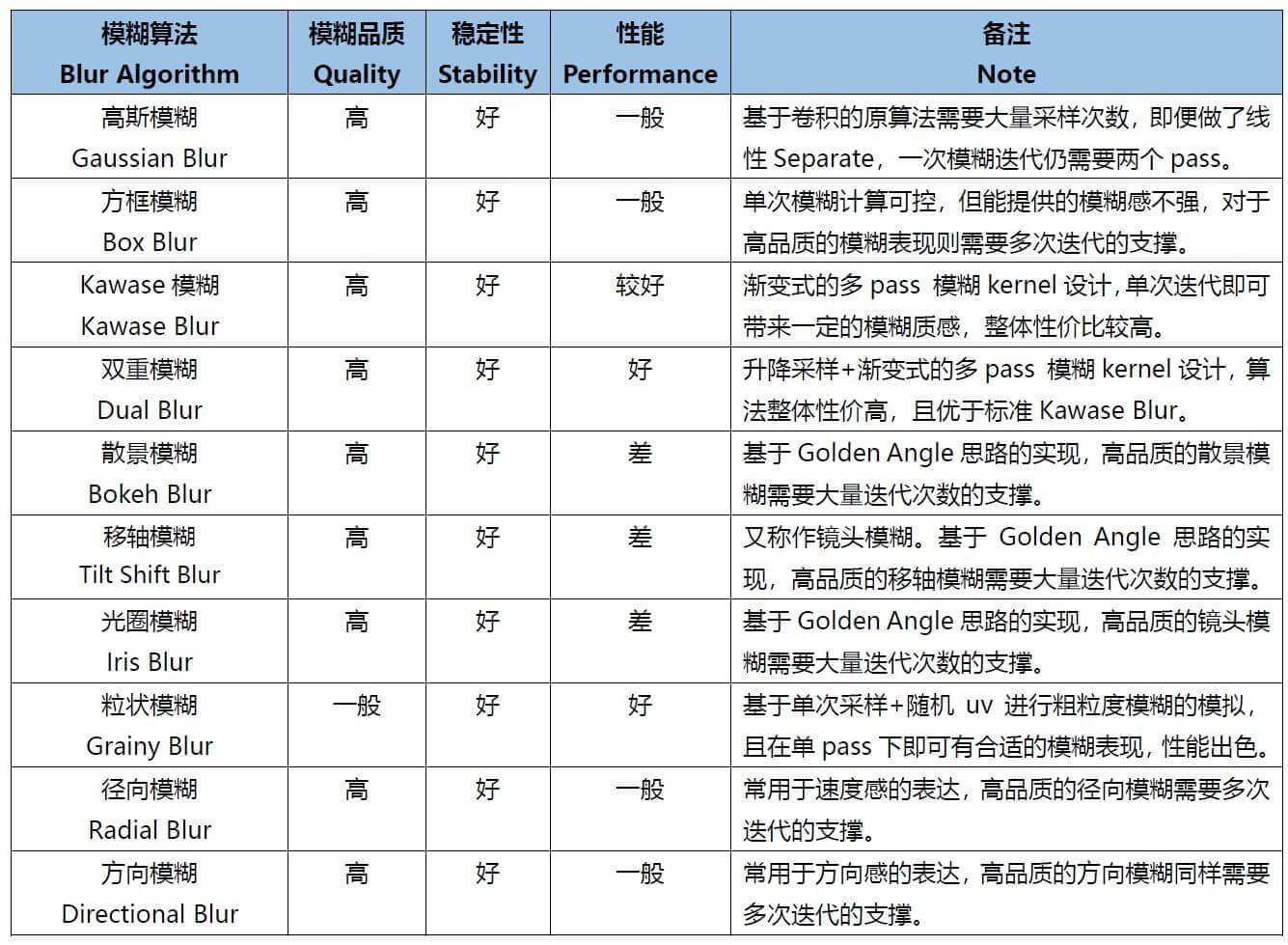### 关于X-PostProcessing Libray

X-PostProcessing Libray，简称XPL，是本人开发的Unity引擎下的高品质开源后处理算法库，旨在提供业界主流的高品质后处理特效的完整解决方案，目前已完美支持Unity Post-processing Stack v2，后续也将提供对Unity引擎URP/LWRP/HDRP的兼容支持。### 一、高斯模糊（Gaussian Blur）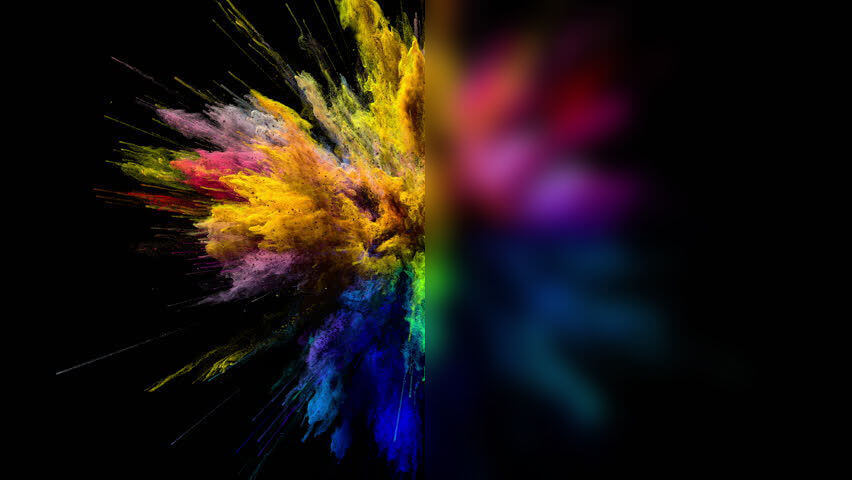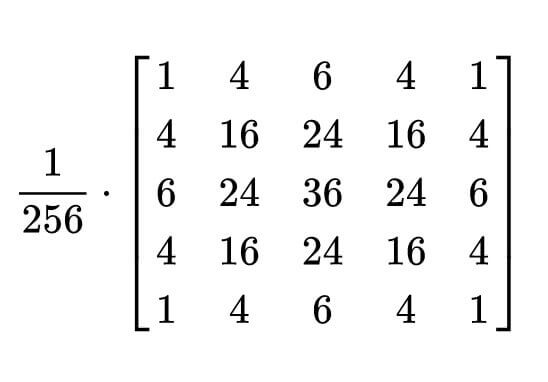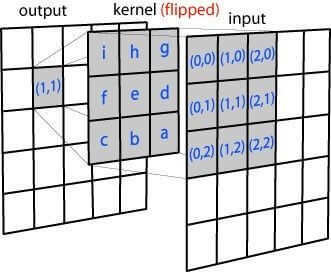N维空间高斯模糊方程可以表示为：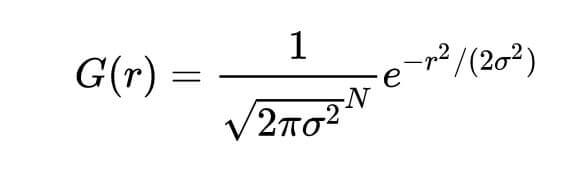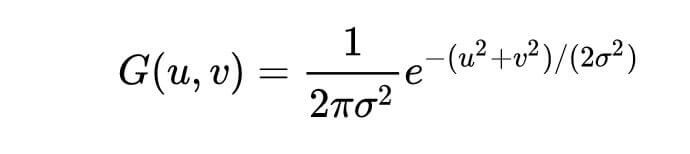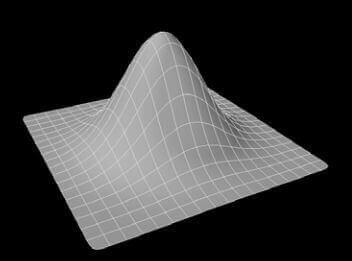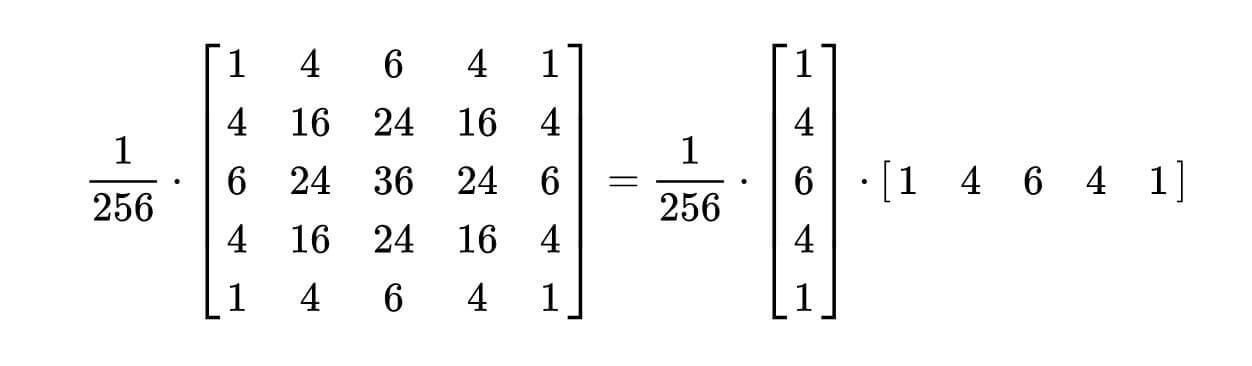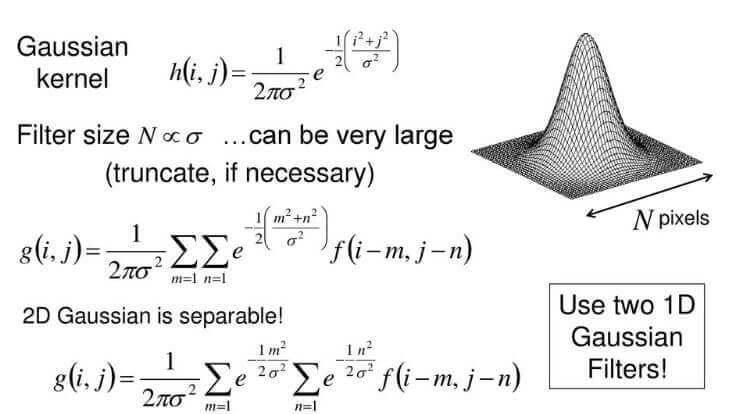``````    float4 FragGaussianBlur(v2f i): SV_Target
{
half4 color = float4(0, 0, 0, 0);

color += 0.40 * SAMPLE_TEXTURE2D(_MainTex, sampler_MainTex, i.uv);
color += 0.15 * SAMPLE_TEXTURE2D(_MainTex, sampler_MainTex, i.uv01.xy);
color += 0.15 * SAMPLE_TEXTURE2D(_MainTex, sampler_MainTex, i.uv01.zw);
color += 0.10 * SAMPLE_TEXTURE2D(_MainTex, sampler_MainTex, i.uv23.xy);
color += 0.10 * SAMPLE_TEXTURE2D(_MainTex, sampler_MainTex, i.uv23.zw);
color += 0.05 * SAMPLE_TEXTURE2D(_MainTex, sampler_MainTex, i.uv45.xy);
color += 0.05 * SAMPLE_TEXTURE2D(_MainTex, sampler_MainTex, i.uv45.zw);

return color;
}
``````

https://github.com/QianMo/X-PostProcessing-Library/tree/master/Assets/X-PostProcessing/Effects/GaussianBlur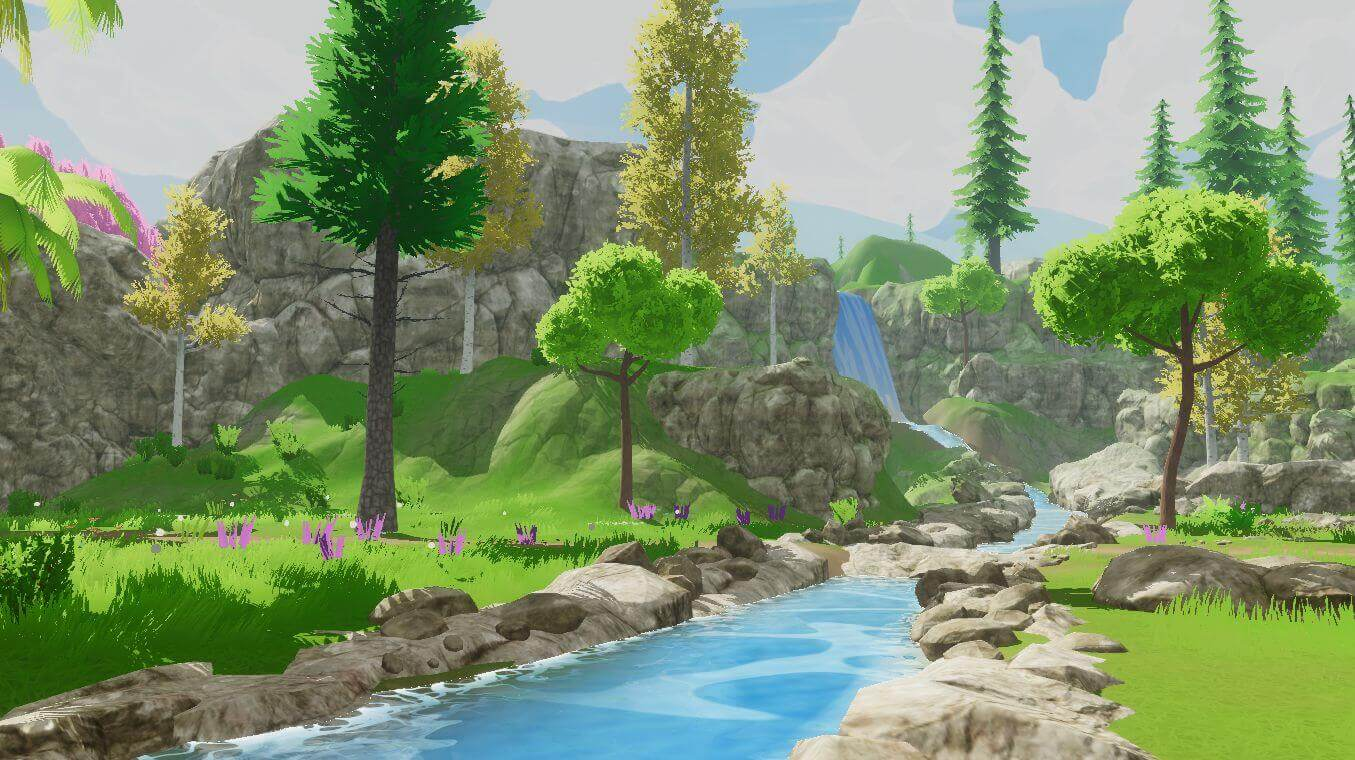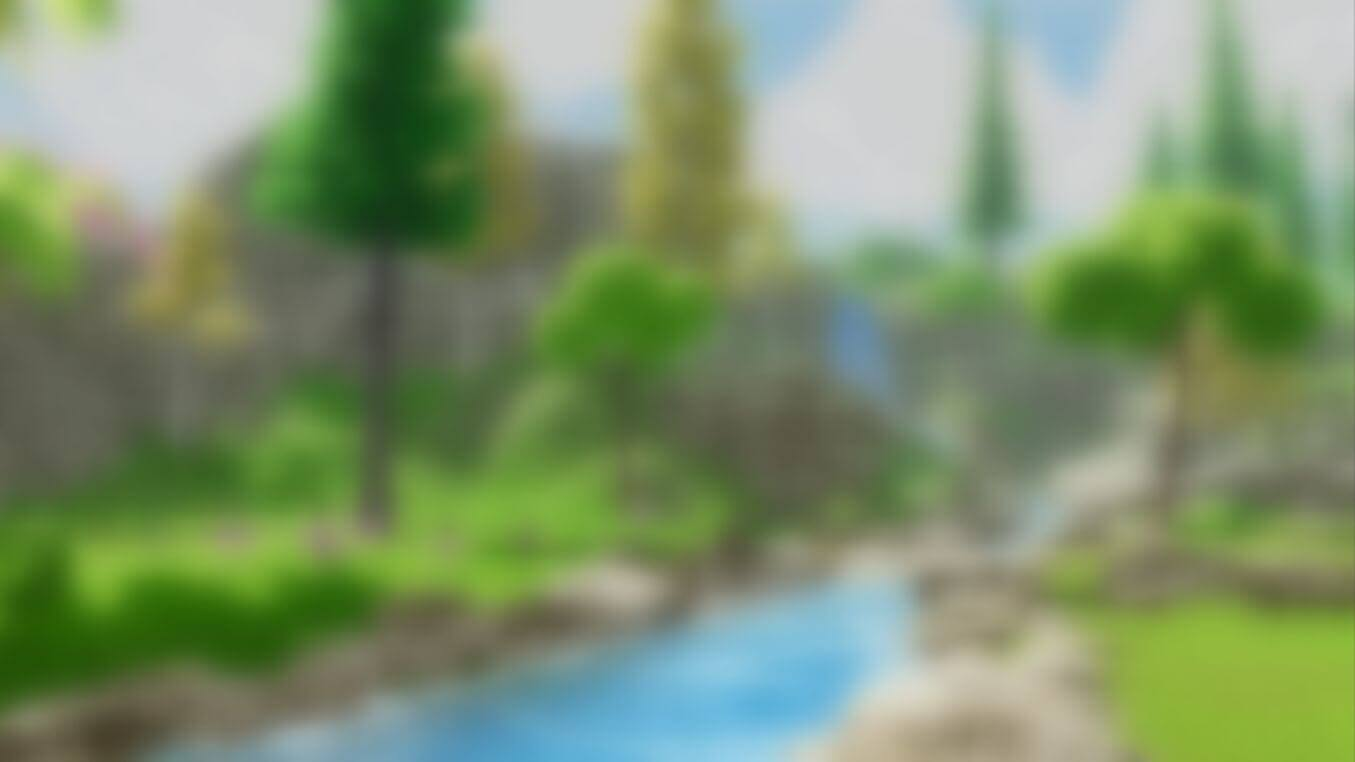### 二、方框模糊（Box Blur）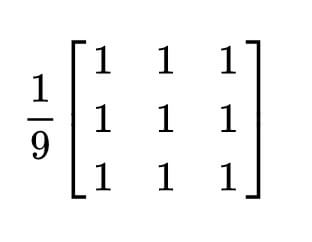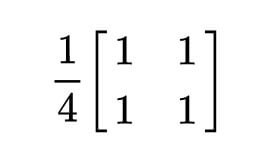Box Blur和高斯模糊的性质对比可见下图：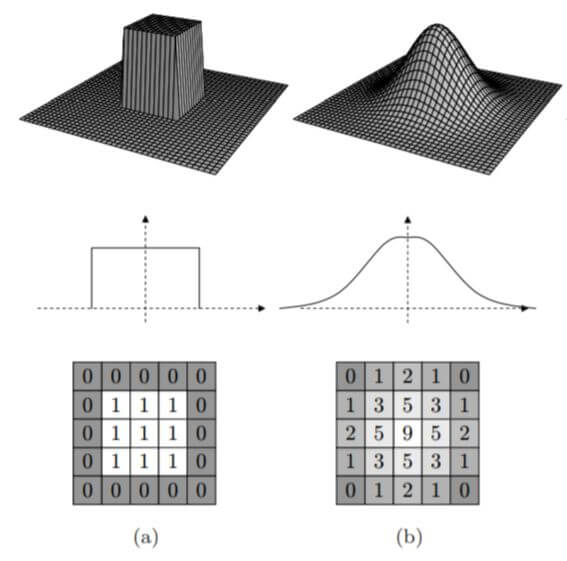3D结构，2D结构和示例矩阵对比（a）Box Blur（b）Gaussian Blur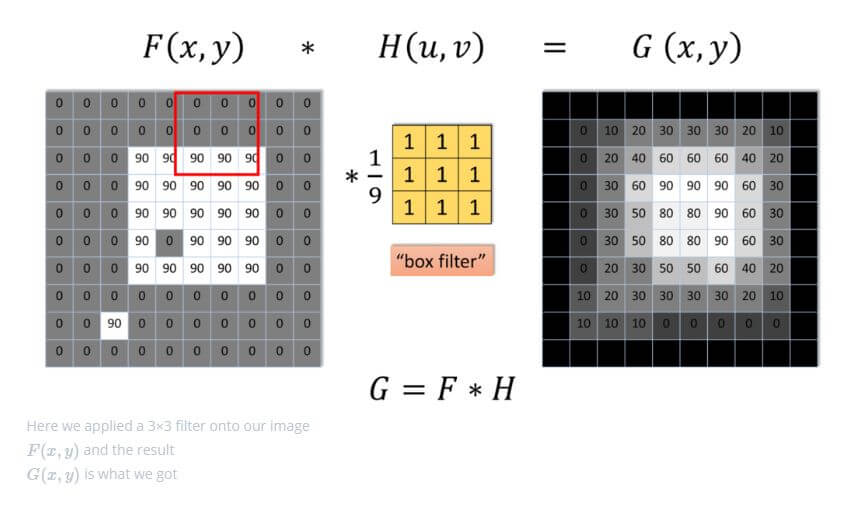Box Blur也是线性可分的，如有需要，也可以借助其此性质，如下所示：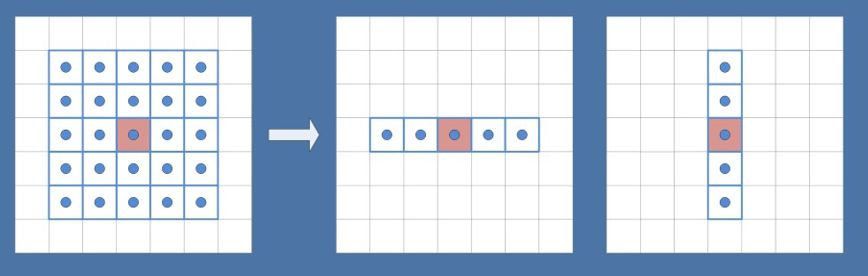https://github.com/QianMo/X-PostProcessing-Library/tree/master/Assets/X-PostProcessing/Effects/TentBlur

``````half4 BoxFilter_4Tap(TEXTURE2D_ARGS(tex, samplerTex), float2 uv, float2 texelSize)
{
float4 d = texelSize.xyxy * float4(-1.0, -1.0, 1.0, 1.0);

half4 s = 0;
s = SAMPLE_TEXTURE2D(tex, samplerTex, uv + d.xy) * 0.25h;  // 1 MUL
s += SAMPLE_TEXTURE2D(tex, samplerTex, uv + d.zy) * 0.25h; // 1 MAD
s += SAMPLE_TEXTURE2D(tex, samplerTex, uv + d.xw) * 0.25h; // 1 MAD
s += SAMPLE_TEXTURE2D(tex, samplerTex, uv + d.zw) * 0.25h; // 1 MAD

return s;
}
``````

https://github.com/QianMo/X-PostProcessing-Library/tree/master/Assets/X-PostProcessing/Effects/BoxBlur

Box Blur的渲染效果接近高斯模糊，但性价比并不高，需要较多的迭代次数才能达到高品质的模糊效果：### 三、Kawase模糊（Kawase Blur）

Kawase Blur于Masaki Kawase 在GDC 2003的分享《Frame Buffer Postprocessing Effects in DOUBLE-S.T.E.A.L (Wreckless)》中提出。Kawase Blur最初用于Bloom后处理特效，但其可以推广作为专门的模糊算法使用，且在模糊外观表现上与高斯模糊非常接近。Kawase Blur的思路是对距离当前像素越来越远的地方对四个角进行采样，且在两个大小相等的纹理之间进行乒乓式的blit。创新点在于，采用了随迭代次数移动的blur kernel，而不是类似高斯模糊，或Box Blur一样从头到尾固定的blur kernel。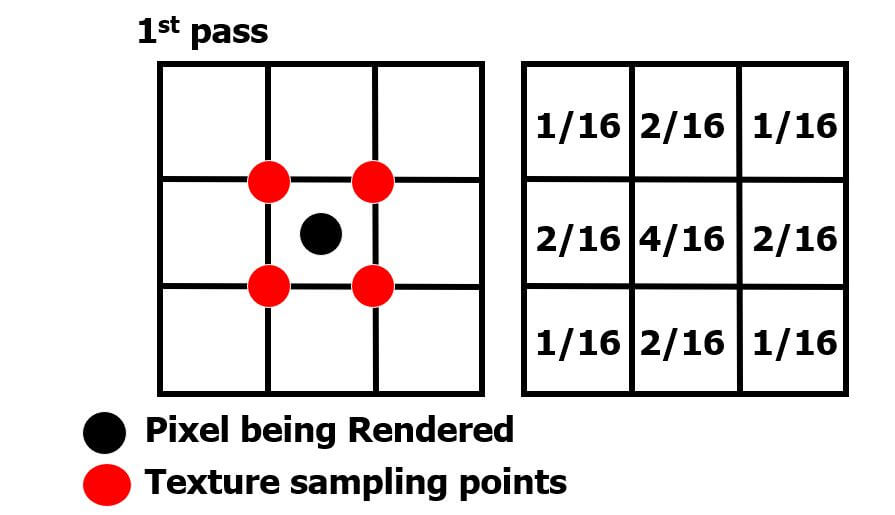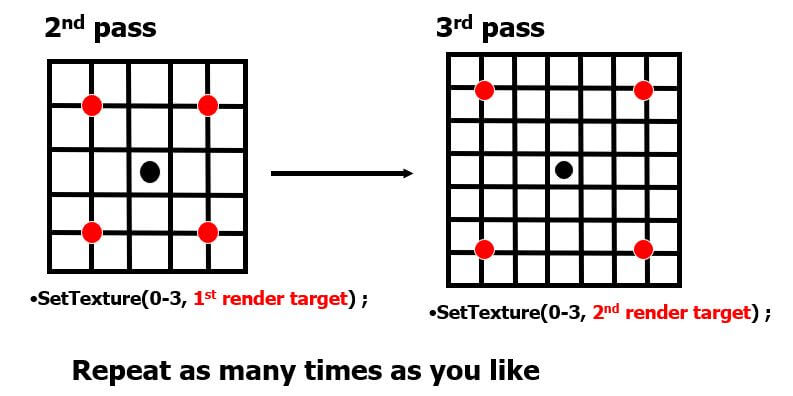``````half4 KawaseBlur(TEXTURE2D_ARGS(tex, samplerTex), float2 uv, float2 texelSize, half pixelOffset)
{
half4 o = 0;
o += SAMPLE_TEXTURE2D(tex, samplerTex, uv + float2(pixelOffset +0.5, pixelOffset +0.5) * texelSize);
o += SAMPLE_TEXTURE2D(tex, samplerTex, uv + float2(-pixelOffset -0.5, pixelOffset +0.5) * texelSize);
o += SAMPLE_TEXTURE2D(tex, samplerTex, uv + float2(-pixelOffset -0.5, -pixelOffset -0.5) * texelSize);
o += SAMPLE_TEXTURE2D(tex, samplerTex, uv + float2(pixelOffset +0.5, -pixelOffset -0.5) * texelSize);
return o * 0.25;
}
``````

https://github.com/QianMo/X-PostProcessing-Library/tree/master/Assets/X-PostProcessing/Effects/KawaseBlur

Kawase Blur渲染效果接近高斯模糊，但具有更好的性能：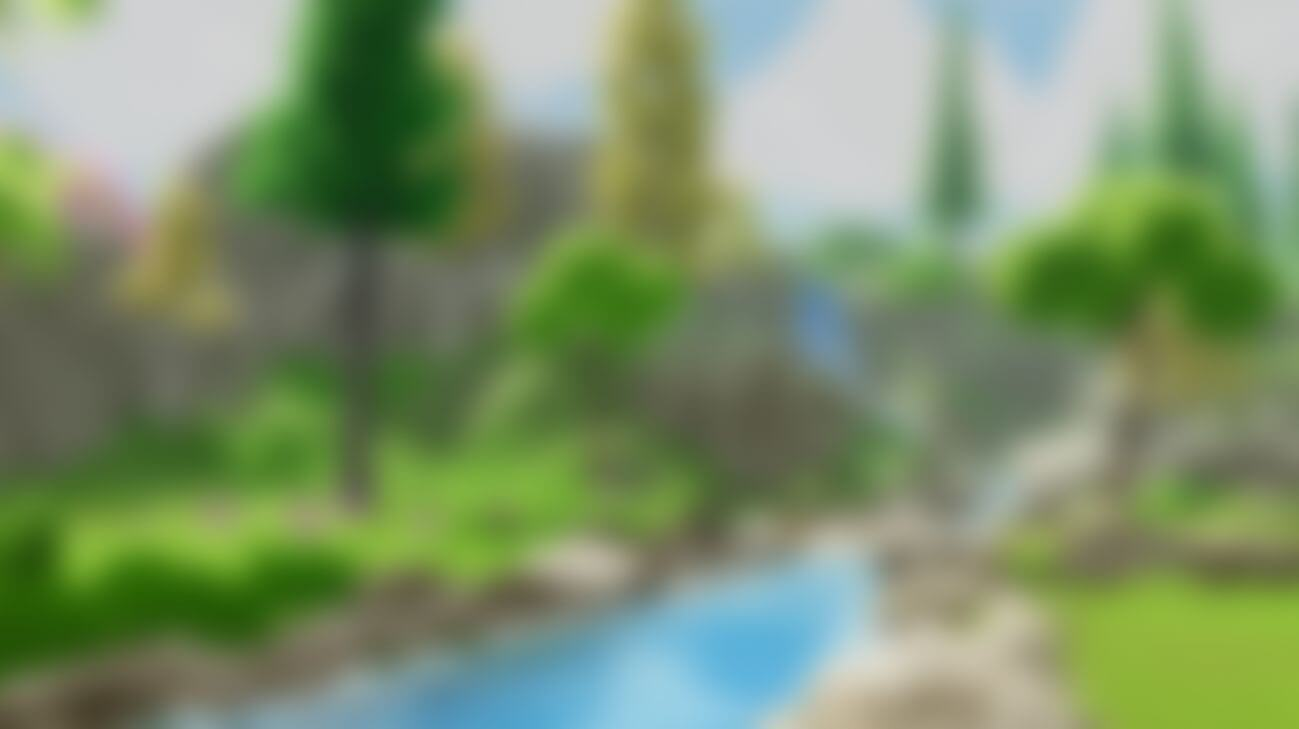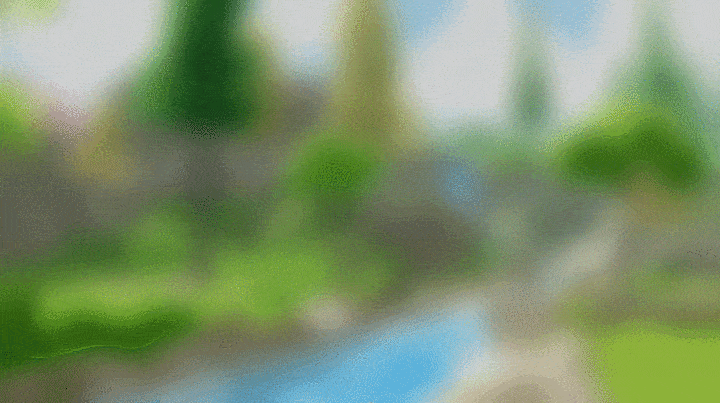### 四、双重模糊（Dual Blur）

Dual Kawase Blur，简称Dual Blur，是SIGGRAPH 2015上ARM团队提出的一种衍生自Kawase Blur的模糊算法。其由两种不同的Blur Kernel构成，如下图所示。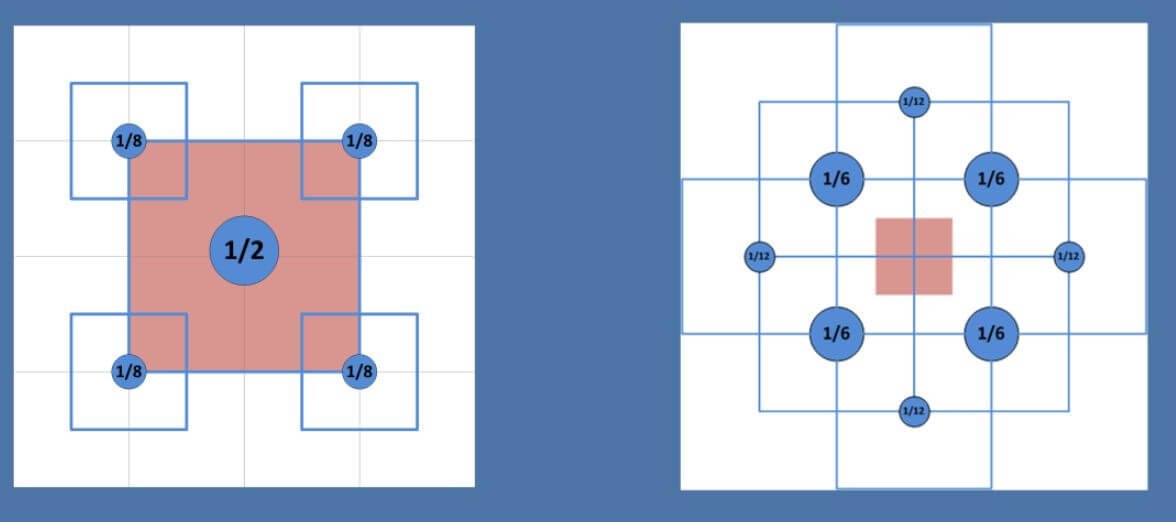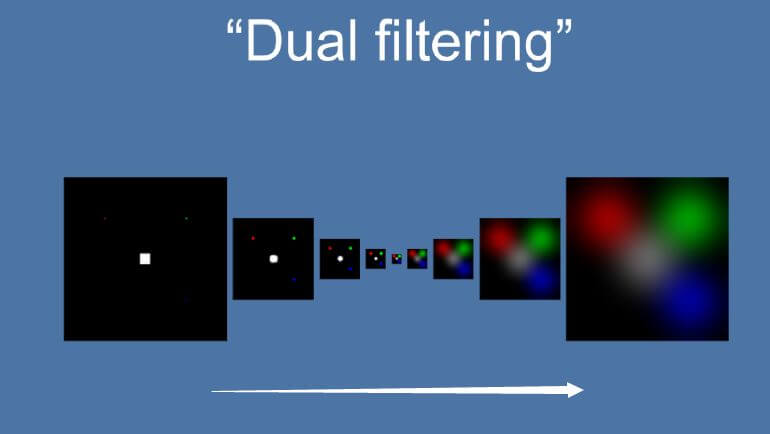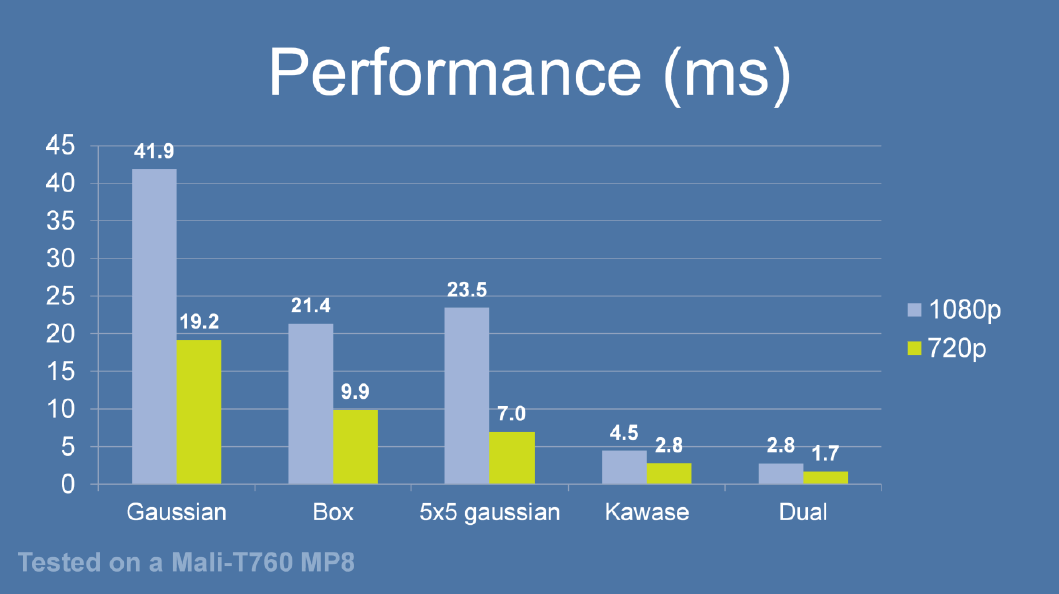``````half4 Frag_DownSample(v2f_DownSample i): SV_Target
{
half4 sum = SAMPLE_TEXTURE2D(_MainTex, sampler_MainTex, i.uv) * 4;
sum += SAMPLE_TEXTURE2D(_MainTex, sampler_MainTex, i.uv01.xy);
sum += SAMPLE_TEXTURE2D(_MainTex, sampler_MainTex, i.uv01.zw);
sum += SAMPLE_TEXTURE2D(_MainTex, sampler_MainTex, i.uv23.xy);
sum += SAMPLE_TEXTURE2D(_MainTex, sampler_MainTex, i.uv23.zw);

return sum * 0.125;
}

half4 Frag_UpSample(v2f_UpSample i): SV_Target
{
half4 sum = 0;
sum += SAMPLE_TEXTURE2D(_MainTex, sampler_MainTex, i.uv01.xy);
sum += SAMPLE_TEXTURE2D(_MainTex, sampler_MainTex, i.uv01.zw) * 2;
sum += SAMPLE_TEXTURE2D(_MainTex, sampler_MainTex, i.uv23.xy);
sum += SAMPLE_TEXTURE2D(_MainTex, sampler_MainTex, i.uv23.zw) * 2;
sum += SAMPLE_TEXTURE2D(_MainTex, sampler_MainTex, i.uv45.xy);
sum += SAMPLE_TEXTURE2D(_MainTex, sampler_MainTex, i.uv45.zw) * 2;
sum += SAMPLE_TEXTURE2D(_MainTex, sampler_MainTex, i.uv67.xy);
sum += SAMPLE_TEXTURE2D(_MainTex, sampler_MainTex, i.uv67.zw) * 2;

return sum * 0.0833;
}
``````

https://github.com/QianMo/X-PostProcessing-Library/tree/master/Assets/X-PostProcessing/Effects/DualKawaseBlur

XPL中也提供了启发自Dual Kawase Blur的Dual Gaussian Blur、Dual Box Blur、Dual Tent Blur的实现。

Dual Kawase Blur最终的模糊效果截图如下，可以看到其与高斯模糊的模糊表现也非常接近：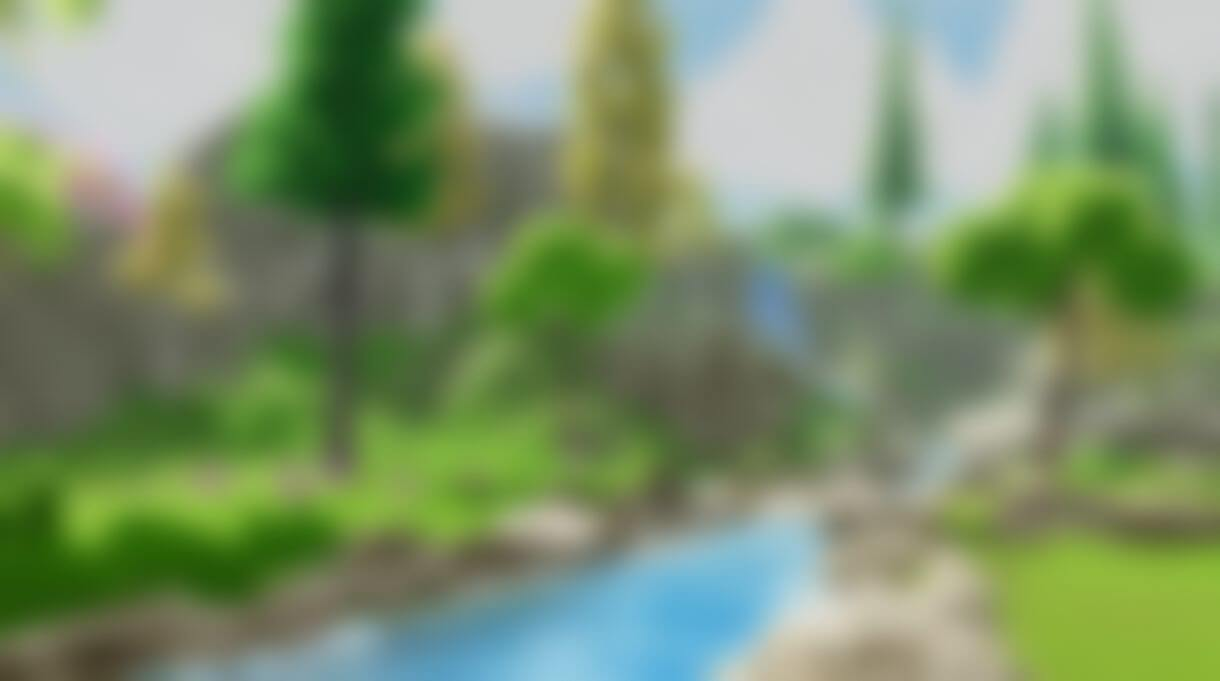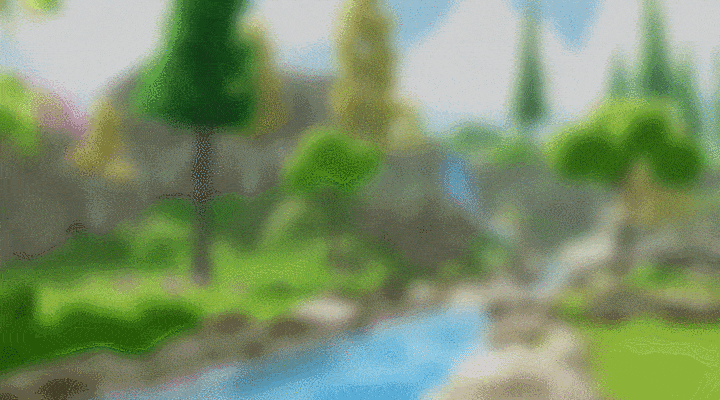### 五、散景模糊（Bokeh Blur）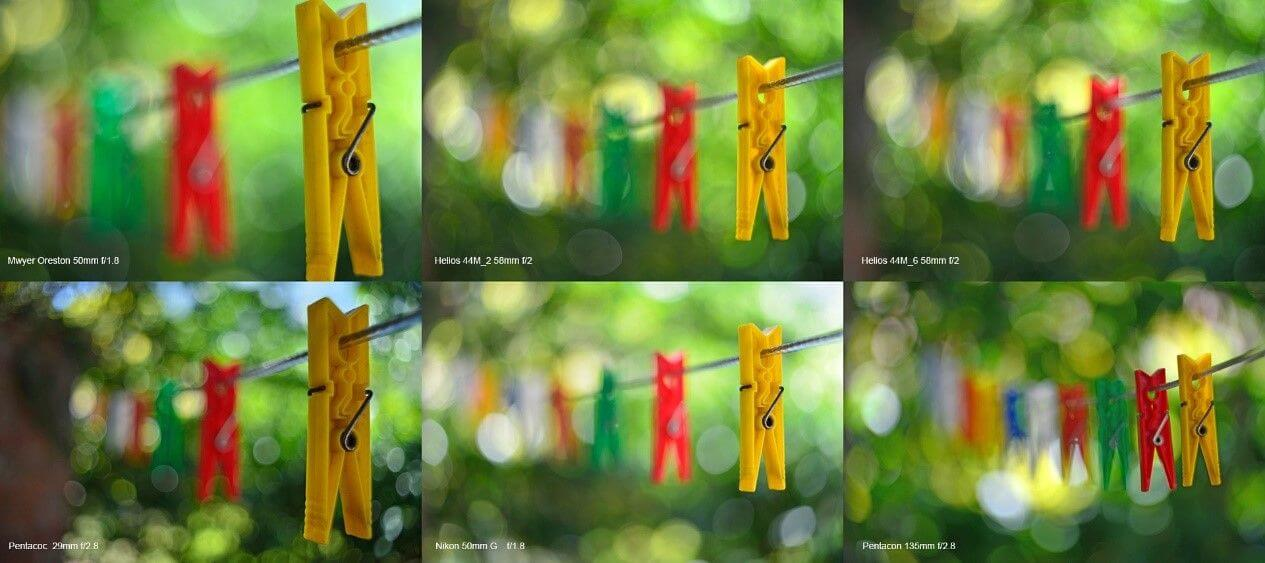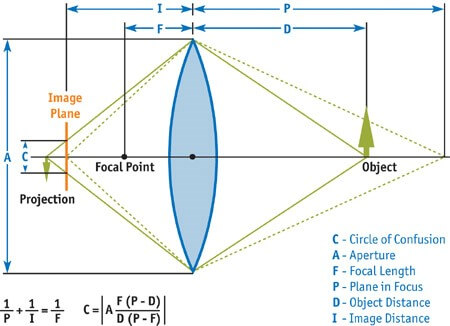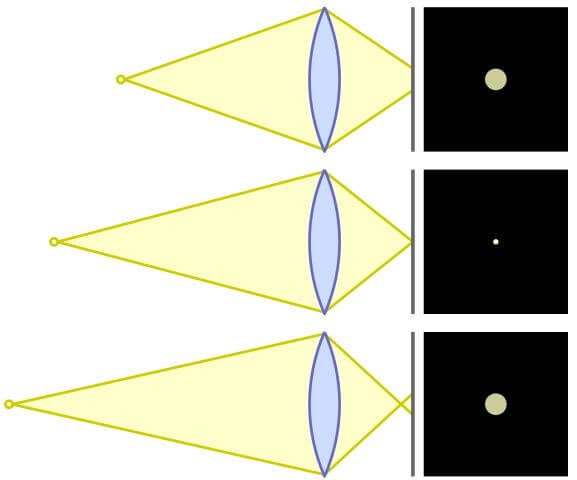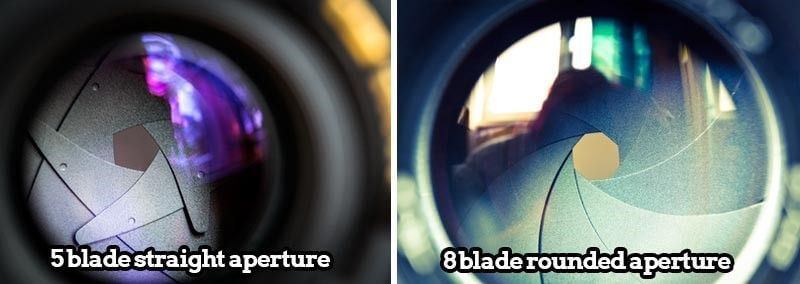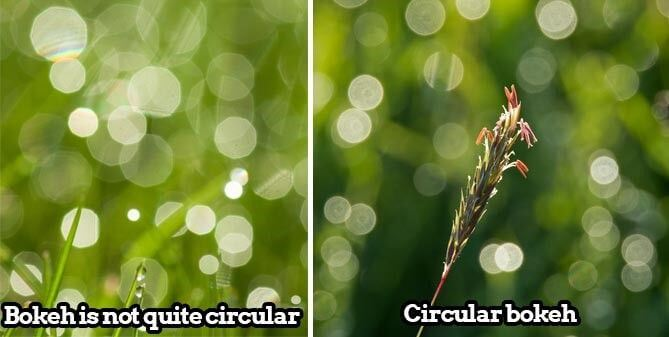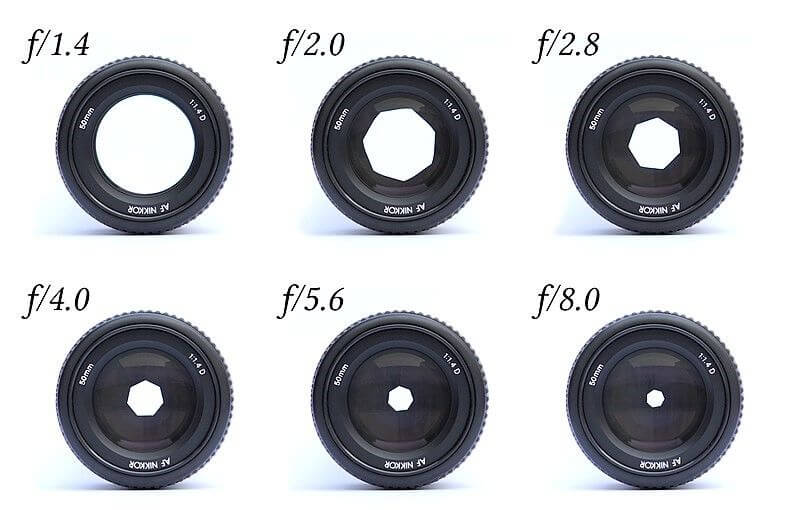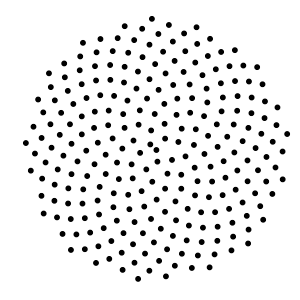``````    half4 BokehBlur(VaryingsDefault i)
{
half2x2 rot = half2x2(_GoldenRot);
half4 accumulator = 0.0;
half4 divisor = 0.0;

half r = 1.0;

for (int j = 0; j < _Iteration; j++)
{
r += 1.0 / r;
angle = mul(rot, angle);
half4 bokeh = SAMPLE_TEXTURE2D(_MainTex, sampler_MainTex, float2(i.texcoord + _PixelSize * (r - 1.0) * angle));
accumulator += bokeh * bokeh;
divisor += bokeh;
}
return accumulator / divisor;
}
``````

https://github.com/QianMo/X-PostProcessing-Library/tree/master/Assets/X-PostProcessing/Effects/BokehBlur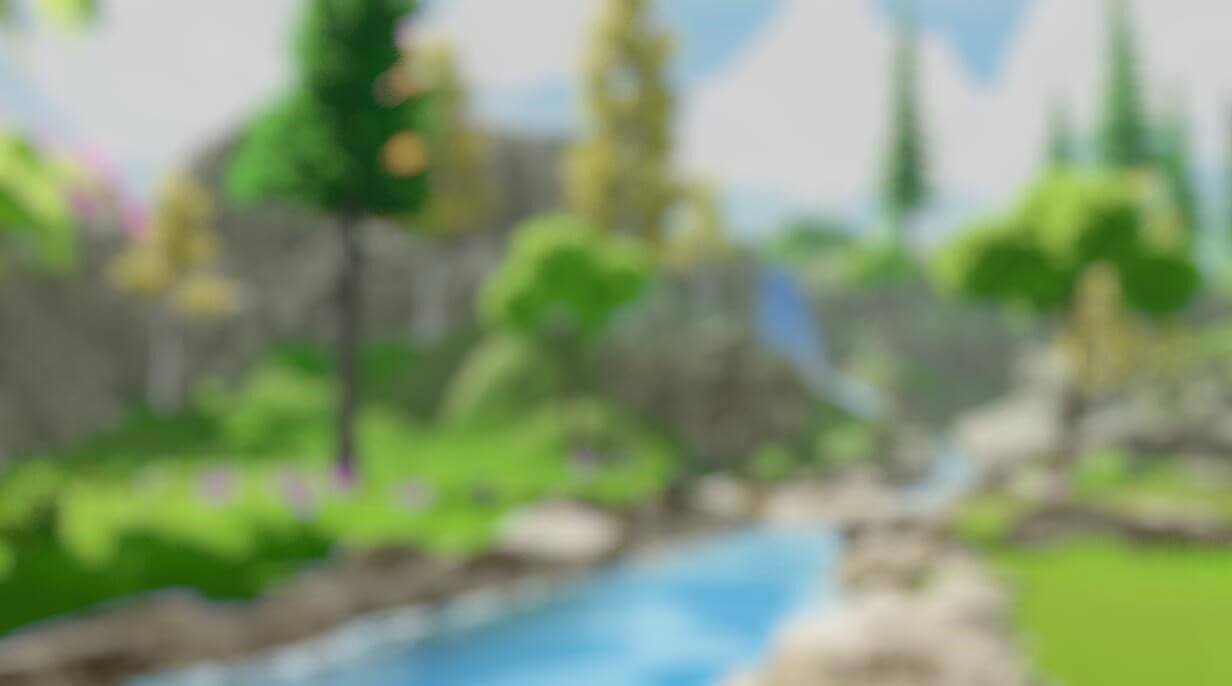### 六、移轴模糊 （Tilt Shift Blur）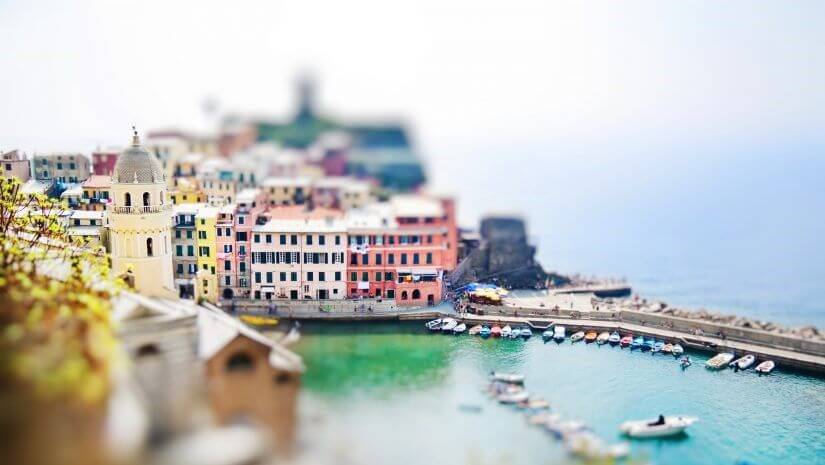``````    float TiltShiftMask(float2 uv)
{
float centerY = uv.y * 2.0 - 1.0 + _Offset; // [0,1] -> [-1,1]
}
``````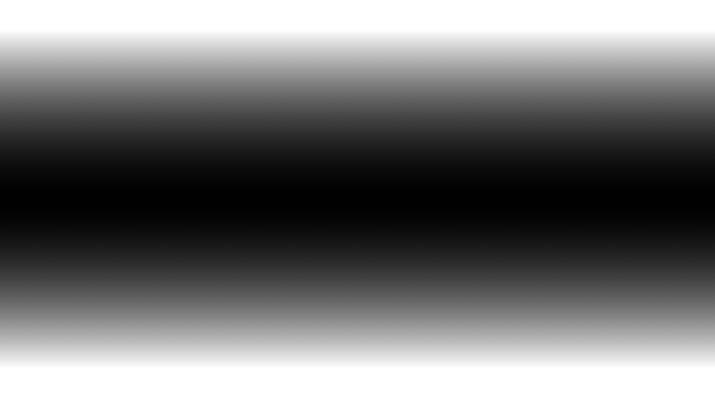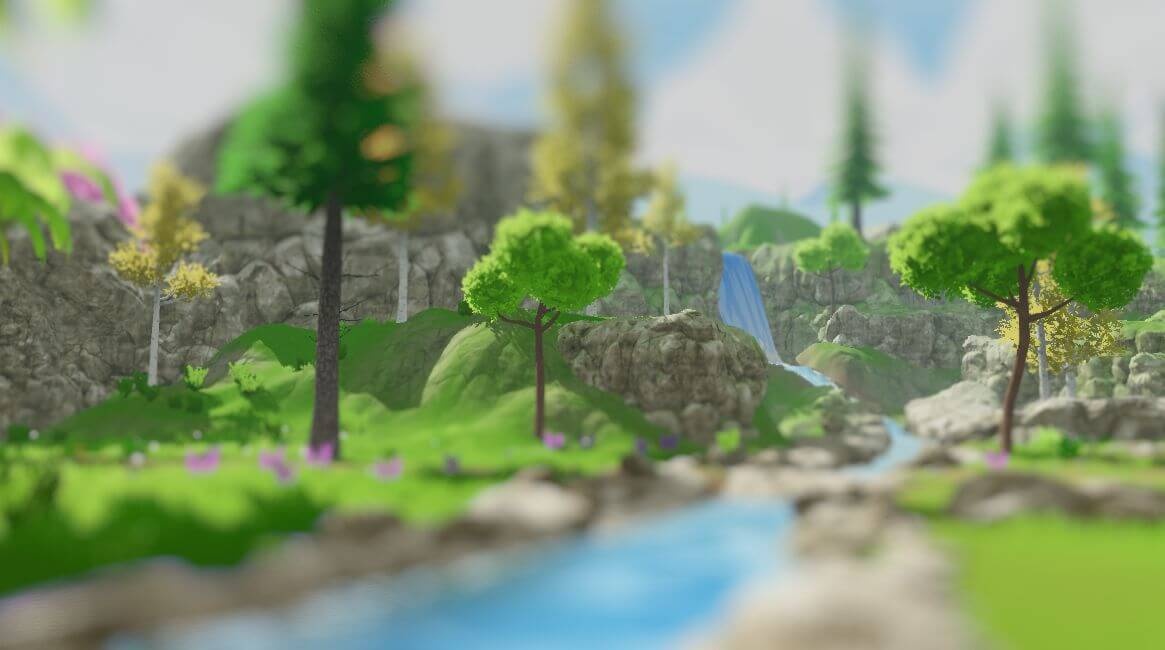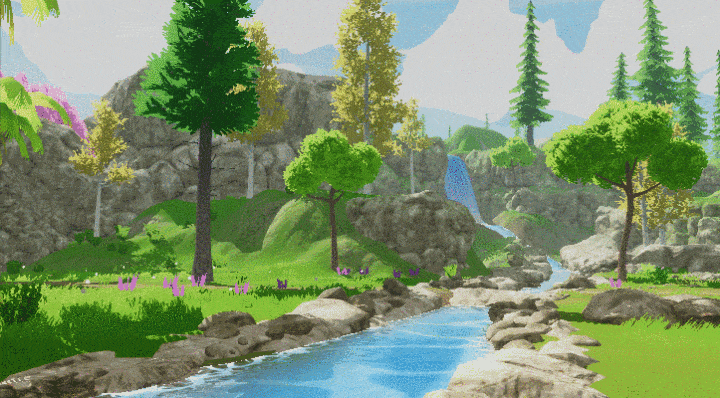### 七、光圈模糊（Iris Blur）

PS：Photoshop中也同样有Tilf-Shift Blur功能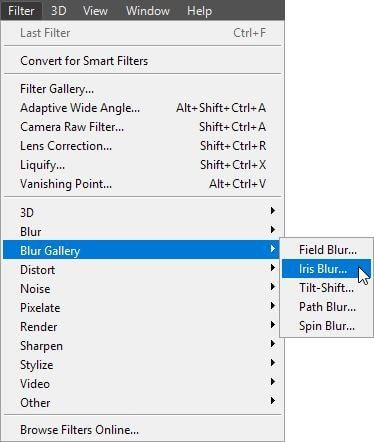Photoshop中的光圈模糊（Iris Blur）功能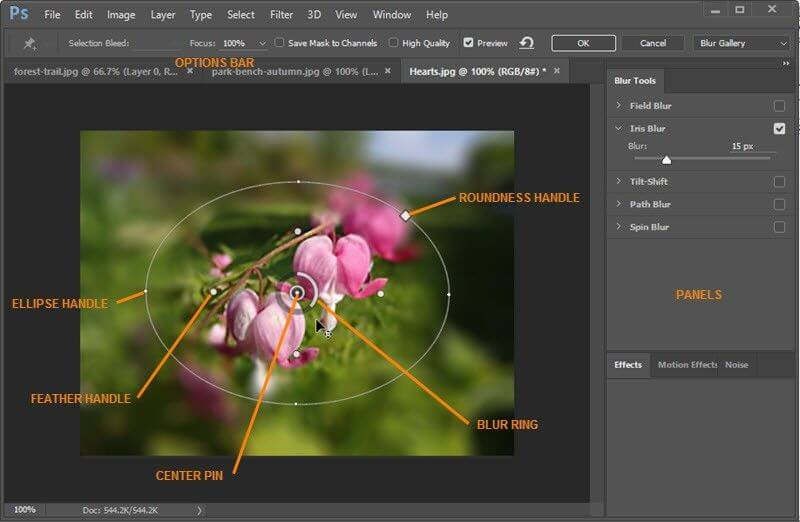Photoshop中的光圈模糊（Iris Blur）功能

``````float IrisMask(float2 uv)
{
float2 center = uv * 2.0 - 1.0 + _Offset; // [0,1] -> [-1,1]
return dot(center, center) * _AreaSize;
}
``````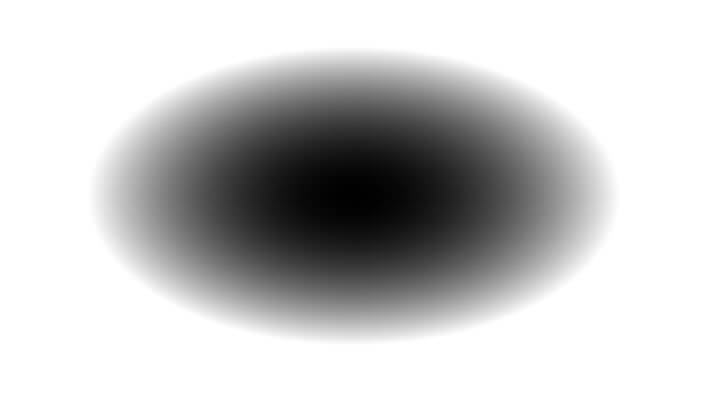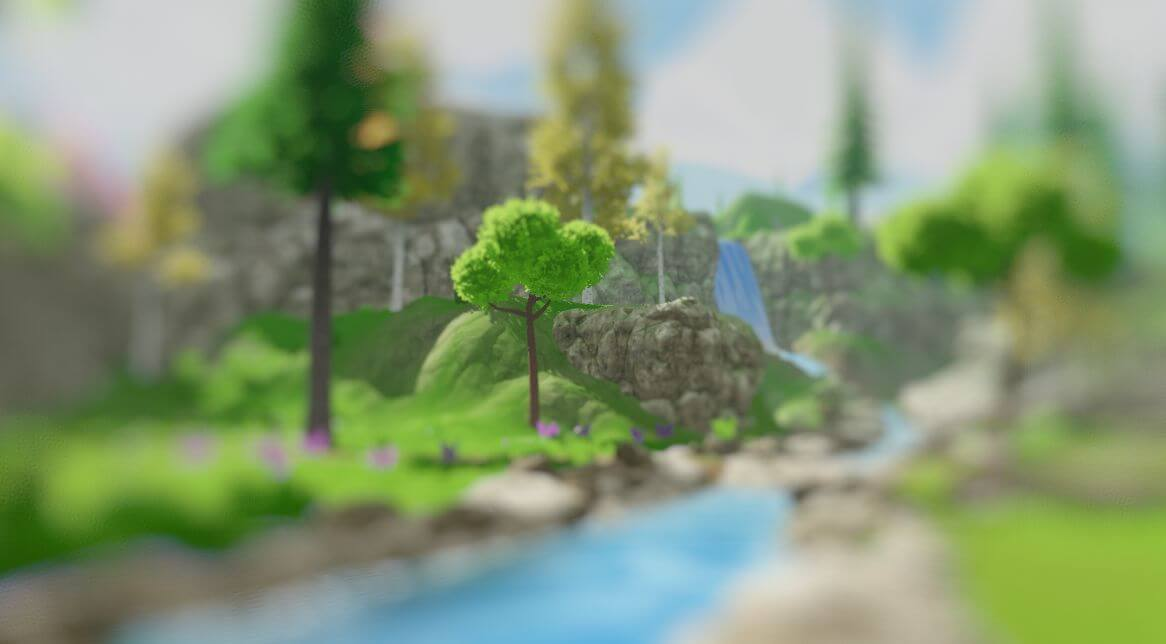https://github.com/QianMo/X-PostProcessing-Library/tree/master/Assets/X-PostProcessing/Effects/IrisBlurV2### 八、粒状模糊（Grainy Blur）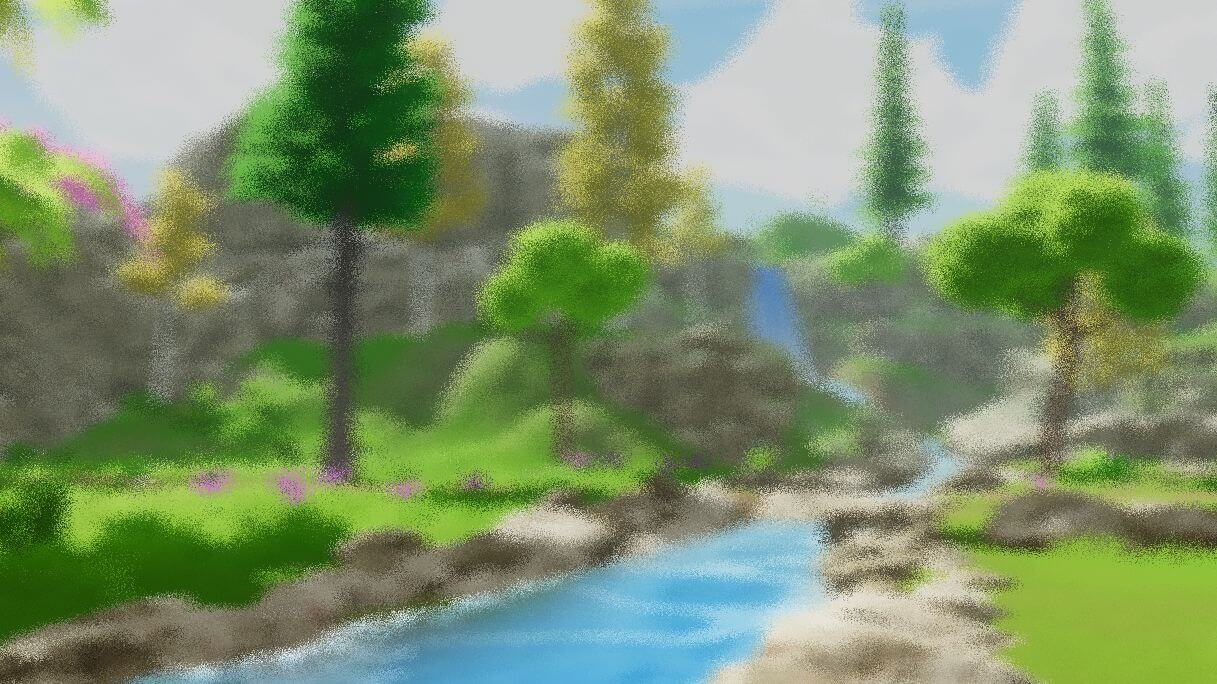``````float Rand(float2 n)
{
return sin(dot(n, half2(1233.224, 1743.335)));
}

half4 GrainyBlur(VaryingsDefault i)
{
half2 randomOffset = float2(0.0, 0.0);
half4 finalColor = half4(0.0, 0.0, 0.0, 0.0);
float random = Rand(i.texcoord);

for (int k = 0; k < int(_Iteration); k ++)
{
random = frac(43758.5453 * random + 0.61432);;
randomOffset.x = (random - 0.5) * 2.0;
random = frac(43758.5453 * random + 0.61432);
randomOffset.y = (random - 0.5) * 2.0;

finalColor += SAMPLE_TEXTURE2D(_MainTex, sampler_MainTex, half2(i.texcoord + randomOffset * _BlurRadius));
}
return finalColor / _Iteration;
}
``````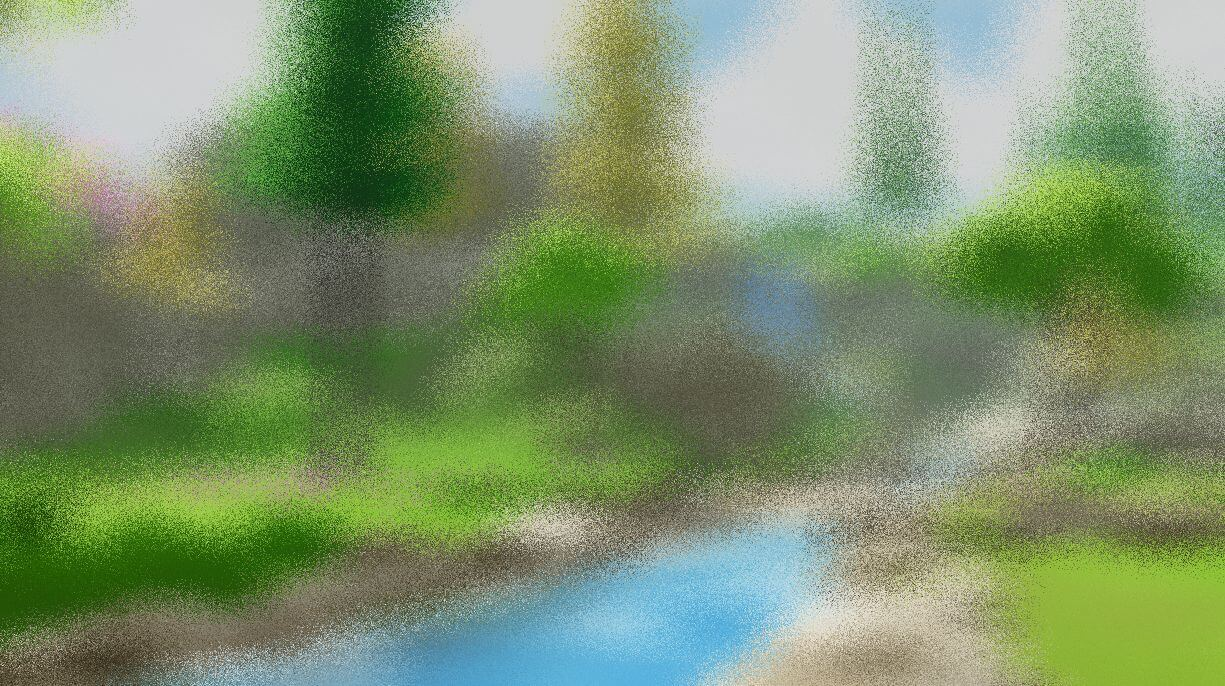https://github.com/QianMo/X-PostProcessing-Library/tree/master/Assets/X-PostProcessing/Effects/GrainyBlur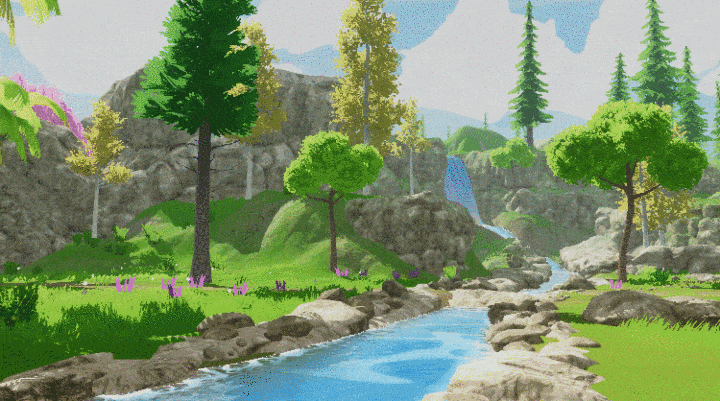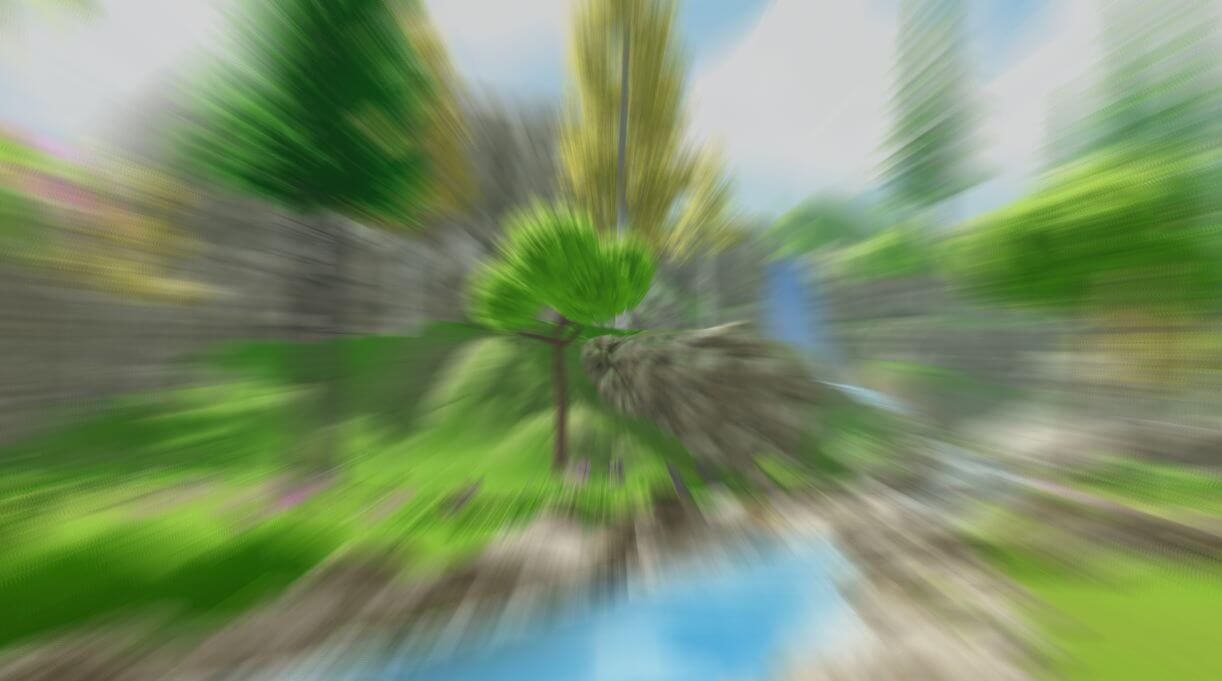``````half4 RadialBlur(VaryingsDefault i)
{

half4 acumulateColor = half4(0, 0, 0, 0);

[unroll(30)]
for (int j = 0; j < _Iteration; j ++)
{
acumulateColor += SAMPLE_TEXTURE2D(_MainTex, sampler_MainTex, i.texcoord);
i.texcoord.xy += blurVector;
}

return acumulateColor / _Iteration;
}
``````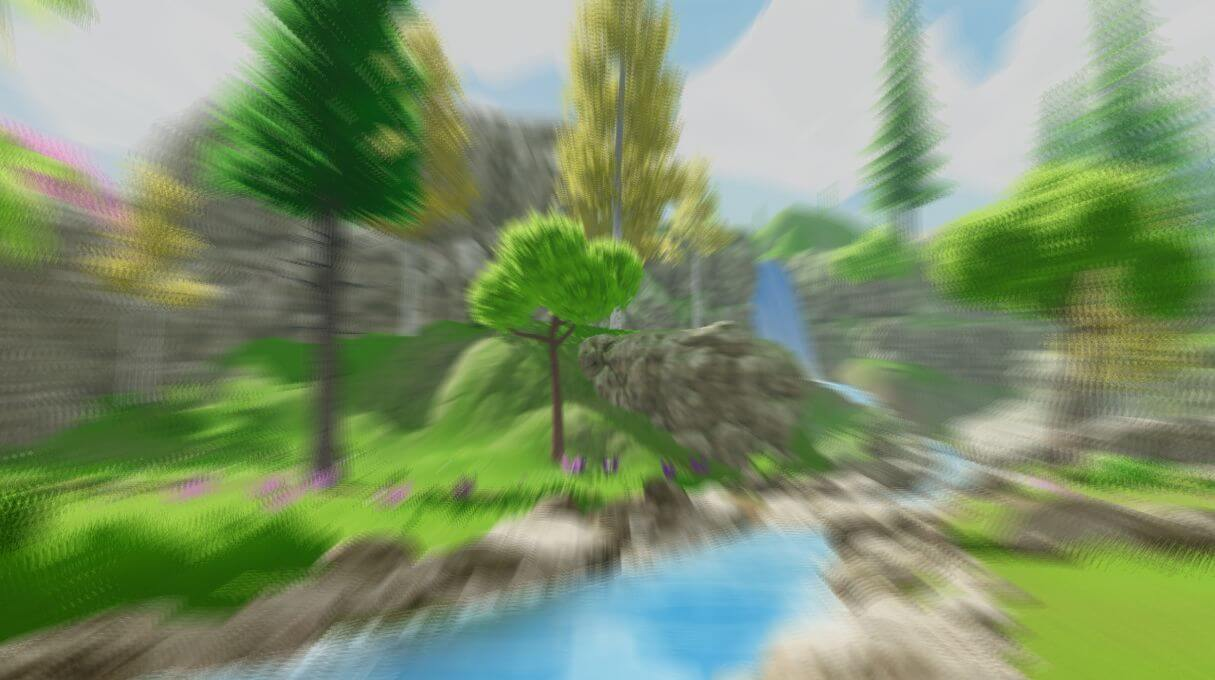### 十、方向模糊（Directional Blur）

``````float sinVal = (Mathf.Sin(settings.Angle) * settings.BlurRadius * 0.05f) / settings.Iteration;
float cosVal = (Mathf.Cos(settings.Angle) * settings.BlurRadius * 0.05f) / settings.Iteration;
``````

``````half4 DirectionalBlur(VaryingsDefault i)
{
half4 color = half4(0.0, 0.0, 0.0, 0.0);

for (int k = -_Iteration; k < _Iteration; k++)
{
color += SAMPLE_TEXTURE2D(_MainTex, sampler_MainTex, i.texcoord - _Direction * k);
}
half4 finalColor = color / (_Iteration * 2.0);

return finalColor;
}
``````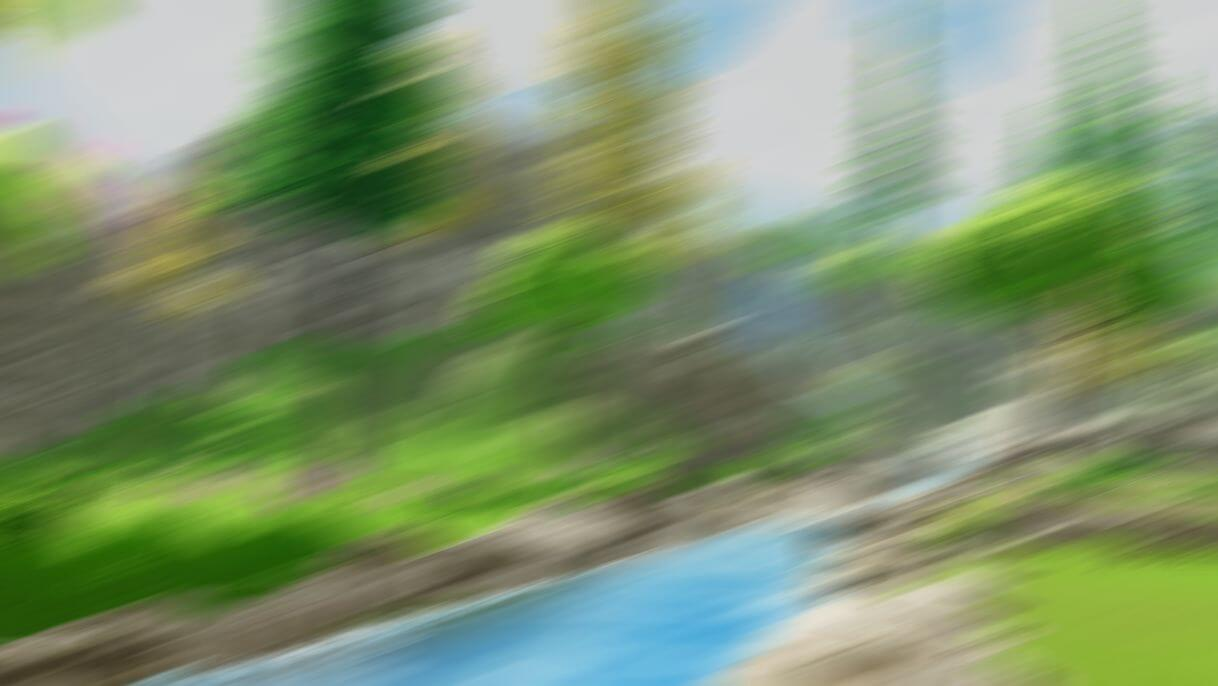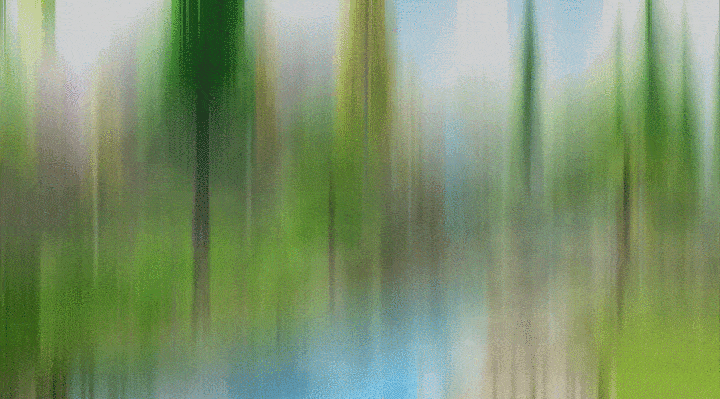### 总结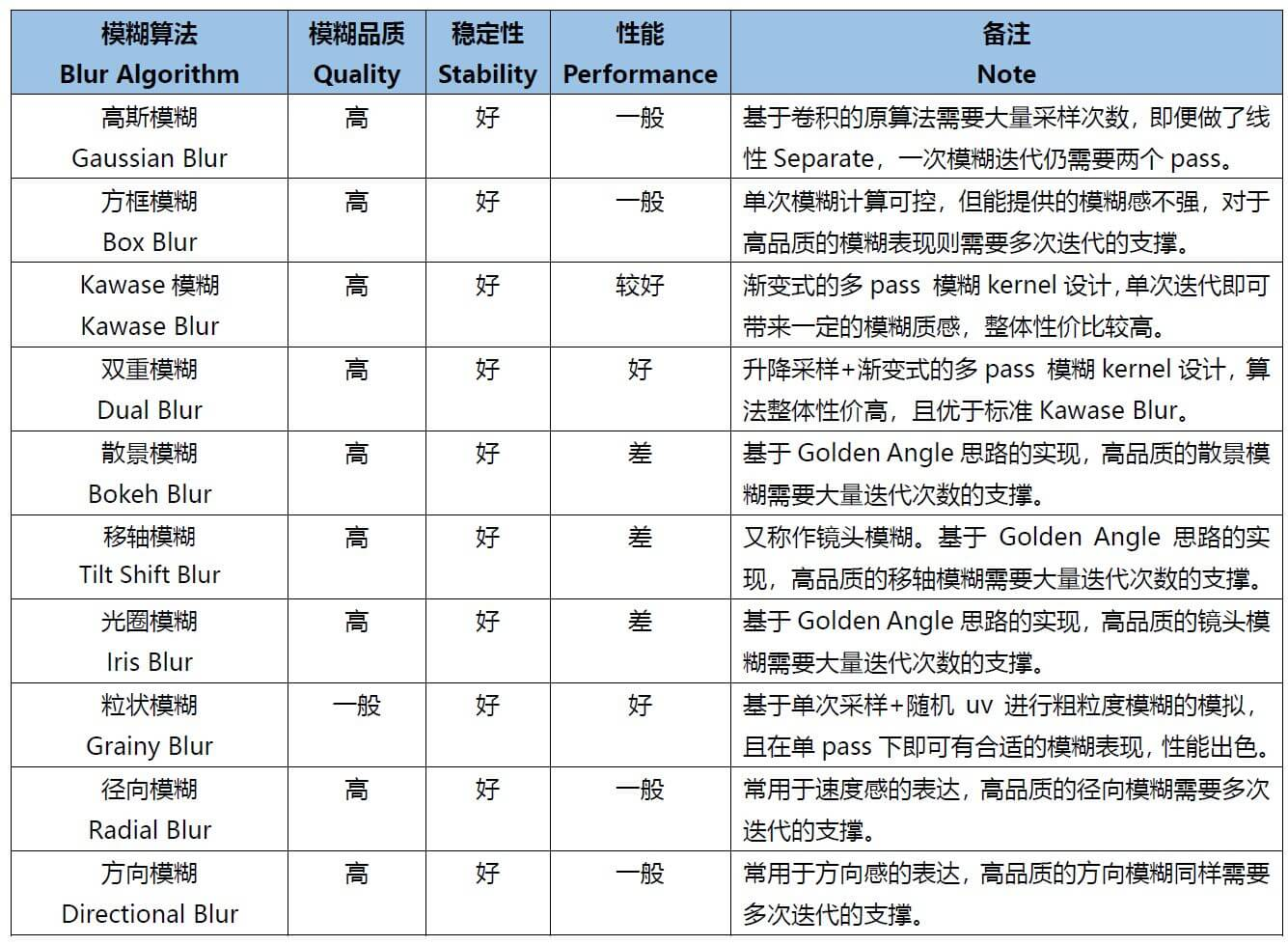Reference
 GDC 2003, Frame Buffer Postprocessing Effects in DOUBLE-S.T.E.A.L (Wreckless)
 SIGGRAPH 2015, Bandwidth-Efficient Rendering
 SIGGRAPH 2001，Fast Image Convolutions
 Gonzalez R C, Woods R E. Digital image processing, 4th edn. ISBN: 9780133356724[J]. 2017.
 http://datahacker.rs/opencv-average-and-gaussian-filter/
 https://towardsdatascience.com/image-processing-class-egbe443-4-filters-aa1037676130
 https://en.wikipedia.org/wiki/Box_blur
 https://en.wikipedia.org/wiki/Gaussian_blur
 http://blog.marmakoide.org/?p=1
 https://developer.nvidia.com/gpugems/gpugems/part-iv-image-processing/chapter-23-depth-field-survey-techniques
 https://www.flickr.com/photos/valpil58/9425151785
 https://en.wikipedia.org/wiki/Bokeh
 https://commons.wikimedia.org/wiki/File:Lenses_with_different_apertures.jpg